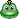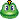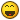## Recommended Posts

At about 40º N. When I hide a cache I take about 9 readings. Then I average. But I still wonder how far off a thousandth is.

And at what latitude would would they both be equal? The equator?

One latitude minute equals one nautical mile equals one quarter mile equals 1852metes. One thousandth latitude minute is thus 1.852 meters (multiply by 39.3700787) equals 72,913.3858268 inches.

As for longitude minutes they are 1852 meters multiplied by the co sinus of the latitude angle.

So at 40deg north one thousandth minute east would be 1,852meters multiplied by cos(40) equals 1,4187143086563473211948311888286 meters multiplied by 39.3700787 equals about 55.854894 inches

cos(40) equals 0,76604444311897803520239265055542

OK?

Edit: Even with spellingverifier typos sneak in.

Edited by baø

Ran it through the Locationless Distance Calculator. Answer 6 feet for latitude.

One latitude minute equals one nautical mile equals one quarter mile ...

One latitude minute does not equal one quarter mile. I assume this was a typo.

0.001 minutes of latitude equals approximately 6.03 feet.

For field measurements, I extend my arms out to the sides.

Fingertip to fingertip is about .001° (lat or lon).

Edited by StarBrand

At your lat it is about 4.5 feet. Here at a lat of 39 it is about 4.65 feet. You are talking about thousands of a minute I take it.

cheers

At about 40º N.  When I hide a cache I take about 9 readings.  Then I average.  But I still wonder how far off a thousandth is.

And at what latitude would would they both be equal?  The equator?

Here's a very handy Table of Lat/Lon Distances that I often refer to.

At your lat it is about 4.5 feet. Here at a lat of 39 it is about 4.65 feet. You are talking about thousands of a minute I take it.

cheers

You are talking about longitude. Please be clear.I just assume about 5 feet. .001=5 ft, .01= 50 ft .1= 500 ft. good enough for an estimate.

What a confusing mess! How about metric.... or is that another thread?I just assume about 5 feet. .001=5 ft, .01= 50 ft .1= 500 ft. good enough for an estimate.

Yeah, I just assume about 5 feet also. You can't get much more accurate then that anyway.

One latitude minute equals one nautical mile equals one quarter mile ...

One latitude minute does not equal one quarter mile. I assume this was a typo.

One quarter mile equals one nautical mile equals 1852 meters. The quarter mile is 1/4 of a sea mile. A sea mile is 7.408 Km. At equator this is 4 distance minutes.

Exactly why a sea mile is 4 minutes I don't know. But this is what I have learned.

One quarter mile equals one nautical mile equals 1852 meters. The quarter mile is 1/4 of a sea mile.  A sea mile is 7.408 Km. At equator this is 4 distance minutes.

Exactly why a sea mile is 4 minutes I don't know. But this is what I have learned.

I thought a standard? mile was 5280 ft and a nautical was like 6080ft? (/3.28 is about 1854 meter?/4= 463 meter in a quarter nautical mile)

And that One nautical mile is a minute of latitude, or a minute of longitute (at the equator, but less towards the poles because of they converge.)

Or am I totally off?

Edited by welch
And that One nautical mile is a minute of latitude, or a minute of longitute (at the equator, but less towards the poles because of they converge.)

Or am I totally off?

No, you are close. But because the earth is not a perfect sphere, both lat and lon distance varies a little from the spheric assumption values. Try this site to see the difference.

Degree of Lat/Lon

That was a good question.

Just to be clear here and quoted from http://en.wikipedia.org/wiki/Nautical_mile "A nautical mile is a unit of length. It is not an SI unit. It is accepted (although discouraged) for use with the SI. The nautical mile is used around the world for maritime and aviation purposes. It is commonly used in international law and treaties, especially regarding the limits of territorial waters. It developed from the geographical mile.

The international standard definition is: 1 nautical mile = 1852 metres (exactly).

Conversions to other units

1 nautical mile of 1852 metres converts to:

1.150 779 45 statute miles

6,076.115 49 feet "

So based on that, .001 of 1 minute of arc (not 1 degree of arc), is about 6 feet. The 6080' per nautical mile is an older British value and is sufficient for most calulations or memoraizingThe original definition of a nautical mile was one minute of latitude. This gave a value easy for sailors to compute and just slightly longer than a land mile. But since the earth is not spherical a nautical mile varies by one's latitude. An average nautical mile can be computed. There were several values for this depending on which datum was used.

The original definition of a meter was one ten millionth of a quadrant of meridian (i.e., 90 degrees of latitude from the equator to the pole). Of course this would depend on the datum used as well and today we have a much better datum than they had when the meter was defined.

If the earth were spherical .001 minutes would equal 1.851851851851... meters!

The modern definition of a nautical mile is 1852 meters exactly.

I like the field method of estimating a difference of .001 minutes.

Stand with your arms extended outward, then fingertip to fingertip is "about" .001 minutes

Edited by Moose Mob
I like the field method of estimating a difference of .001 minutes.

Stand with your arms extended outward, then fingertip to fingertip is "about" .001 minutes

That is a good field method for general estimations. A fingertip to fingertip measurement is also exactly the same as a persons height, BTW.

I like the field method of estimating a difference of .001 minutes.

Stand with your arms extended outward, then fingertip to fingertip is "about" .001 minutes

That is a good field method for general estimations. A fingertip to fingertip measurement is also exactly the same as a persons height, BTW.

Yep I realized that the vast majority of adults are between 5 and 6 feet tall, and that .001 was 5-6 foot in the mid latitudes. It's difficult to stop and make the height (vertical) to horizontal distance cooralation, to the fingertip to fingertip cooralation was much easier to think about.

And the first knuckle to second nuckle is about and inch. But that takes the thread off topic, doesn't it?

## Join the conversation

You can post now and register later. If you have an account, sign in now to post with your account.
Note: Your post will require moderator approval before it will be visible.×   Pasted as rich text.   Paste as plain text instead

Only 75 emoji are allowed.